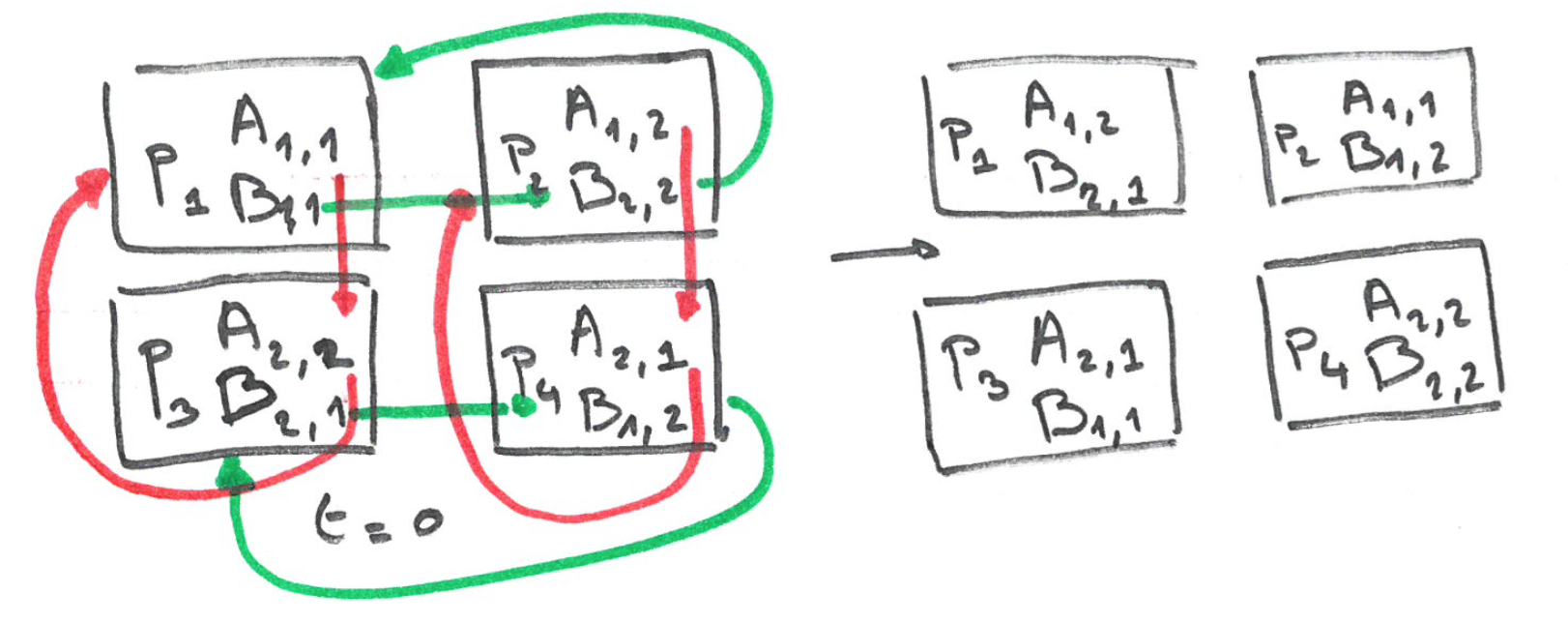Blog Post

# Distributed Matrix product

Matrix multiplication is a mathematical operation that takes two matrices and produces another one as a result. Before explaining how distributed matrices product can work, we are going to do a quick reminder of what is a matrix and what's a dot product of matrices. You can skip that part if everything is fresh in your mind.

## Matrices and dot product

### Matrices

A matrix is a data structure that's made of columns and rows. It is basically a Tic tac toe grid filled with numbers and a potentially infinite number of rows and columns.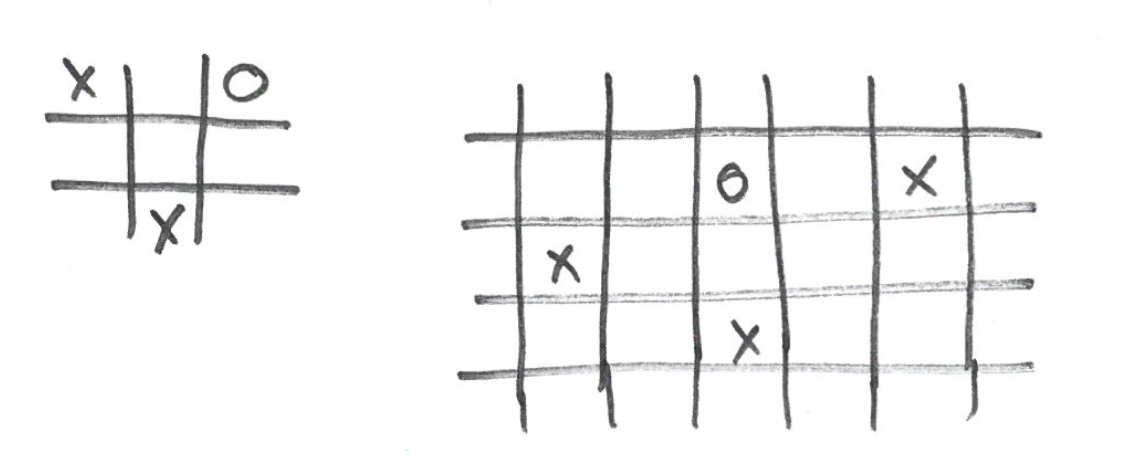When a matrix has the same number of rows and columns we say that it is squared ... because it looks like a square.### Dot Product

A dot product is an operation that takes two matrices and returns another matrix as the result. Let's take an example to go through the process.

Let A be a squared 2x2 (2 rows & 2 columns) matrix and B another squared 2x2 matrix.This matrix product will result in another 2x2 matrix CIn that configuration each element of C: c(1,1), c(1,2), c(2,1) and c(2,2) is the result of sums and products on the corresponding rows of A and columns of B with the following fashion:

• c(1,1) = A(1,1) _ B(1,1) + A(1,2) _ B(2,1) = 1 _ 2 + 3 _ 3 = 11
• c(1,2) = A(1,1) _ B(1,2) + A(1,2) _ B(2,2) = 1 _ 1 + 3 _ 1 = 4
• c(2,1) = A(2,1) _ B(1,1) + A(2,2) _ B(2,1) = 5 _ 2 + 2 _ 3 = 16
• c(2,2) = A(2,1) _ B(1,2) + A(2,2) _ B(2,2) = 5 _ 1 + 2 _ 1 = 7

The overall operation look like this, in red is circled the elements of the matrix involved in getting the c(1,1) = 11 part of the resulting matrix C: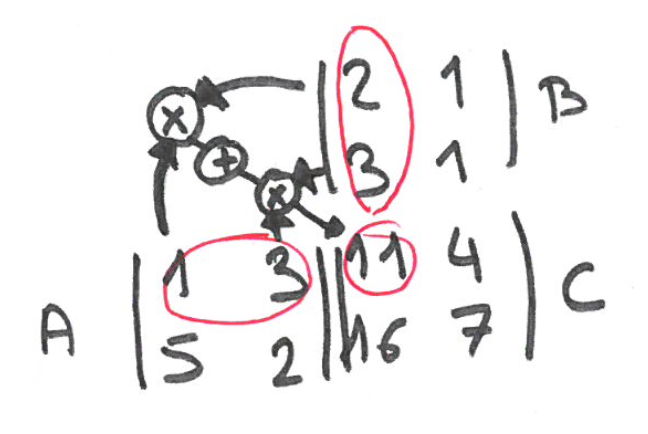## Distributed matrix product

Why trying to distribute this operation across processors in a computer?

This operation is used in a lot of Machine Learning algorithms. The more data you have in machine learning, the better the output of your algorithm is likely to be. So we want to be able to make that operation on very large matrices that potentially don't fit into memory.

For the sake of simplicity we will consider squared matrices but everything can be generalized to all kind of matrices.

### Column/Row data segmentation

Since our main problem is to be able to store the whole matrices data into memory on one processor we will have to distribute it.

Let p be the number of processors we can use.

A first way to do that is to split the first matrix in p slices of rows and split B in p slices of columns.Let's attribute each slice of the data for each matrix to a processor. What happens in processor 1 ?

It gets the first rows slice of A and the first column slice of B. With that, and using the definition of the dot product, it is only able to compute a specific part of C, the top left corner of C.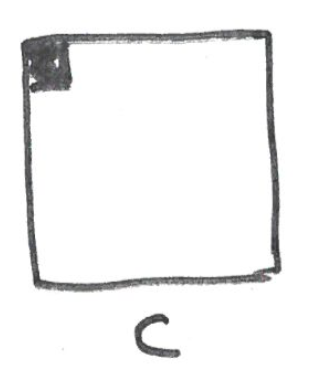Following that logic, each processor will be able to compute a part of the diagonal on C. Resulting in a partial result of the dot product for C. The only elements that we will be able to compute will the the blacked squared elements on the following drawing.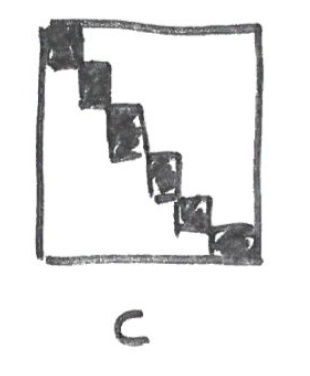In order to fill the blanks we need to do several iterations in which we will change how the slice of B are affected to processors. Now, processor 1 will have rows' slice 1 of A, columns slice 2 of B, and will be able to compute the corresponding part of C.

The intersection of slice 1 and 2. If we imagine all the processors in a ring, all each processor has to do at each iteration is pass to the right their current slice of B to their neighbor until everybody has seen all the slices of B.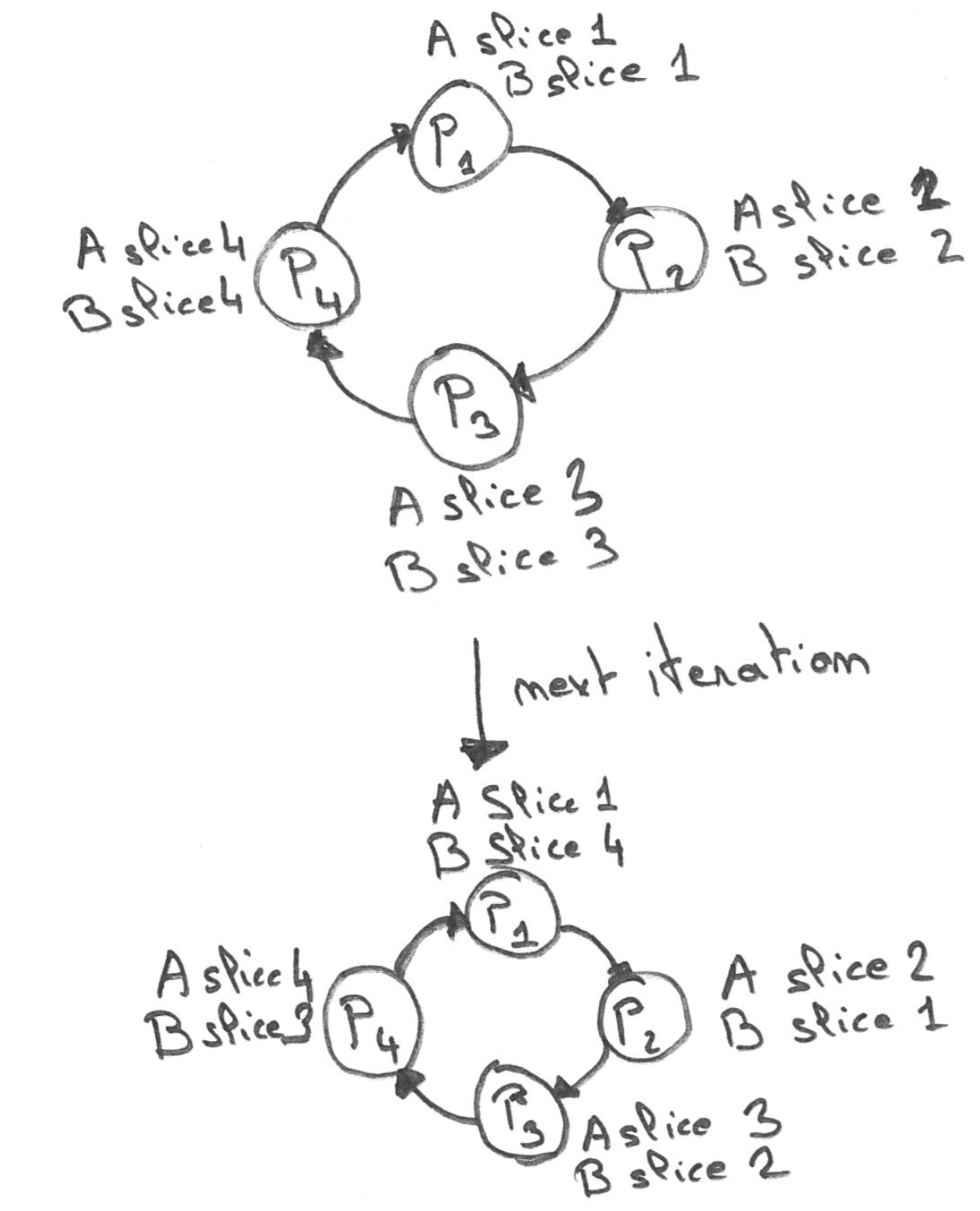The result will of the next iteration will be the parts of C drawn in red.This really gives us the intuition that if we repeat that operation enough we will be able to compute the whole matrix by collecting all the small part that we have computed.

### Submatrices data segmentation

An intuition that you can get from the previous method is that instead of slicing matrices vertically and horizontally by the number of processors, could we slice it in p little squares, p submatricesIn that configuration, each one of the c(x,x) can be expressed as the sum of the dot product of a subset of A & B submatrices.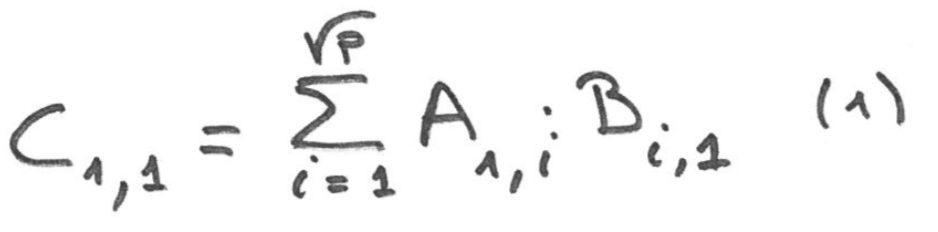That equation expresses the fact that, like before, we still need the entire row and column to compute the complete dot product for a square but the way to get there is different. Now, each processor is responsible for computing only one subpart of the resulting matrix C and doesn't need whole rows or columns to do so.

Now what's needed to get the result of that computation ?

Let's take processor 1 as an example, with a total of 4 processors. Then, in order to compute its submatrix of C, C(1,1), it needs A(1,1), B(1,1), A(1,2) and B(1,2):

• C(1,1) = A(1,1) B(1,1) + A(1,2)B(2,1)
• C(1,2) = A(1,1)B(1,2) + A(1,2)B(2,2)
• C(2,1) = A(2,1)B(1,1) + A(2,2)B(2,1)
• C(2,2) = A(2,1)B(1,2) + A(2,2)B(2,2)

In order to have all those pieces we are going to play the same game as in the previous algorithm. We are going to swap around the pieces of A and B so that every processor gets what it needs without having all the data at once.

This time let's imagine the processors in a 2x2 grid. On each iteration, each processor is going to give its part of B to the processor one way down and its part of A one way right. In the following drawing, A parts follow the the green arrows and B parts the red arrows.